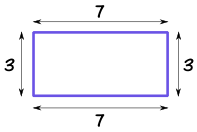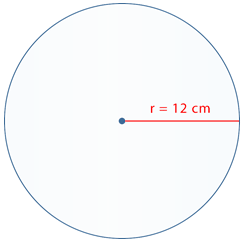# What I learned in grade 9 surface area

What is surface area?                                                                                                                                    Surface area is the area of the surface of a 2-d or 3-d shape. Area is how much of a surface is showing.

What is the difference between surface area and perimeter?

The difference is perimeter is measuring the length around the outside of a shape, and are is measuring the surface of a shape.

Perimeter of a rectangle: You just add up all the sidesSo in this example you would just go 7+7+3+3= 20 so your perimeter would be 20. (Keep in mind to add units, in this example there are none but if there are add them)

Area of a rectangle: Base times heightSo in this example you would just go 7 times 3= 21 so your area would be 21.  (Keep in mind to add units, in this example there are none but if there are add them). Area is always units squared or cubed so if your answer was 21cm it would be 21cm2.

A good way to know whether you square the area or cube it is if its a 2-d shape its to the power of two and if its a 3-d you put it to the power of three.

Surface area of a triangle:

The formula for calculating a triangles surface area is base times height divided by two. This is because two triangles make a square so if you just calculate it as you would for a square then all you have to do is divide it by two.So in this example you would times 10 (the height) and 12 (the base) 10 times 12 equals 120. So now all you have to do is divide that number by two. 120 divided by 2 =60 >Your finale answer is 60.= two triangles

Surface area of a circle:

When calculating the area of a circle you should use the formula pie times radius squared. Radius is the length from the centre of the circle to one of the edges and diameter is the length from one end of a circle to another. (So when finding radius you can just divide the diameter by two and when finding to diameter you can just times the radius by two).      Pie=  π  (3.1415926535).So in this example you would just do π times 12 squared which equals 37.7cm2

The surface area of a cube:

To find the area of a 3-d cube you should just find the measurement of one cube then multiply it by the number of squares.So in this equation if a= 1cm you would know the area of one square is one so you just times one by the number of squares showing. In this equation there are six squares so all you do is 1 times 6 which equals six so your area is 6cm3 .

Finding the Area of 2-d Irregular shapes:When you look at this you probably think its going to be really hard to find the area. Well if you look really closely it can be split into three shapes (a square and two rectangles). Whenever you see an irregular shape first look to see if it can be broken up into separate shapes and if it can then just find the area of each of those shapes and add them all up.